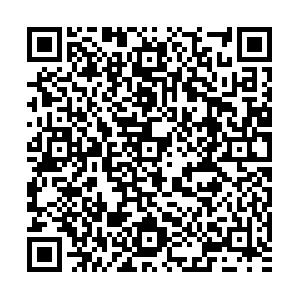# Squeezed back-to-back correlations of K+K−in d + Au collisions at ${\sqrt{{s_{NN}}}}$=200 GeV and Au + Au collisions at ${\sqrt{{s_{NN}}}}$= 62.4 GeV

• We investigate the squeezed back-to-back correlations (BBC) of $K^+$$K^-$, caused by the mass modification of particles in the dense medium formed in d + Au collisions at $\sqrt{s_{NN}} = 200$GeV and Au + Au collisions at $\sqrt{s_{NN}} = 62.4$GeV. Considering that some kaons may not be affected by the medium, we further study the BBC functions of $K^+$$K^-$when parts of all kaons have a mass-shift. Our results indicate that the BBC functions of $K^+$$K^-$can be observed when only ~10% of all kaons have a mass-shift in d + Au collisions at $\sqrt{s_{NN}} = 200$GeV and the peripheral collisions of Au + Au at $\sqrt{s_{NN}} = 62.4$GeV. Since the BBC function is caused by the mass-shift due to the interactions between the particle and the medium, the successful detection of the BBC function indirectly marks that the dense medium has formed in these collision systems. We suggest the experimental measurement of the BBC function of $K^+$$K^-$in d + Au collisions at $\sqrt{s_{NN}} = 200$GeV and peripheral collisions of Au + Au at $\sqrt{s_{NN}} = 62.4$GeV.
••Get Citation
Yong Zhang, Jing Yang and Weihua Wu. Squeezed back-to-back correlations of K +K in d + Au collisions at ${\sqrt{{s_{NN}}}}$=200 GeV and Au + Au collisions at ${\sqrt{{s_{NN}}}}$= 62.4 GeV[J]. Chinese Physics C, 2019, 43(7): 074105-1-074105-9. doi: 10.1088/1674-1137/43/7/074105
Yong Zhang, Jing Yang and Weihua Wu. Squeezed back-to-back correlations of K +K in d + Au collisions at ${\sqrt{{s_{NN}}}}$=200 GeV and Au + Au collisions at ${\sqrt{{s_{NN}}}}$= 62.4 GeV[J]. Chinese Physics C, 2019, 43(7): 074105-1-074105-9.Milestone
Article Metric

Article Views(936)
Cited by(0)
Policy on re-use
To reuse of subscription content published by CPC, the users need to request permission from CPC, unless the content was published under an Open Access license which automatically permits that type of reuse.
###### 通讯作者: 陈斌, bchen63@163.com
• 1.

沈阳化工大学材料科学与工程学院 沈阳 110142

Title:
Email:

## Squeezed back-to-back correlations of K+K−in d + Au collisions at ${\sqrt{{s_{NN}}}}$=200 GeV and Au + Au collisions at ${\sqrt{{s_{NN}}}}$= 62.4 GeV

###### Corresponding author: Jing Yang, yangjdut@gmail.com
• 1. School of Mathematics and Physics, Jiangsu University of Technology, Changzhou, Jiangsu 213001, China
• 2. School of Science,Inner Mongolia University of Science & Technology, Baotou,Inner Mongolia Autonomous Region 014010, China
• 3. School of Physics and School of International Education Teachers, Changchun Normal University, Changchun 130032, China

Abstract: We investigate the squeezed back-to-back correlations (BBC) of $K^+$$K^-$, caused by the mass modification of particles in the dense medium formed in d + Au collisions at $\sqrt{s_{NN}} = 200$GeV and Au + Au collisions at $\sqrt{s_{NN}} = 62.4$GeV. Considering that some kaons may not be affected by the medium, we further study the BBC functions of $K^+$$K^-$when parts of all kaons have a mass-shift. Our results indicate that the BBC functions of $K^+$$K^-$can be observed when only ~10% of all kaons have a mass-shift in d + Au collisions at $\sqrt{s_{NN}} = 200$GeV and the peripheral collisions of Au + Au at $\sqrt{s_{NN}} = 62.4$GeV. Since the BBC function is caused by the mass-shift due to the interactions between the particle and the medium, the successful detection of the BBC function indirectly marks that the dense medium has formed in these collision systems. We suggest the experimental measurement of the BBC function of $K^+$$K^-$in d + Au collisions at $\sqrt{s_{NN}} = 200$GeV and peripheral collisions of Au + Au at $\sqrt{s_{NN}} = 62.4$GeV.

### HTML关注分享DownLoad:  Full-Size Img  PowerPoint